Function Repository Resource:

# SpanningTreeCount

Count the number of labeled spanning trees in a graph

Contributed by: Wolfram Staff (original content by Sriram V. Pemmaraju and Steven S. Skiena)
 ResourceFunction["SpanningTreeCount"][g] gives the number of labeled spanning trees of the graph g.

## Details and Options

A spanning tree of a graph g is a tree whose vertex set includes all the vertices of g and whose edges are all contained in the edge set of g.
In a labeled tree, each vertex is given a unique label.

## Examples

### Basic Examples (1)

Find the number of spanning trees of a graph:

 In:=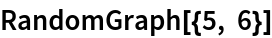Out=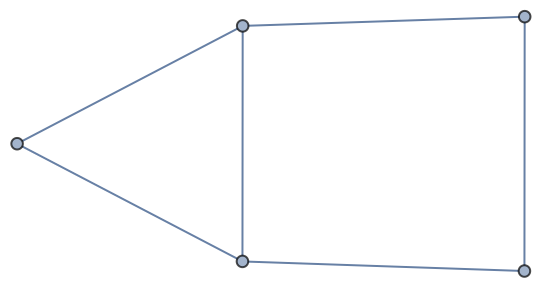In:=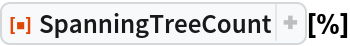Out=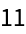### Properties and Relations (4)

A tree contains exactly one spanning tree:

 In:=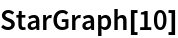Out=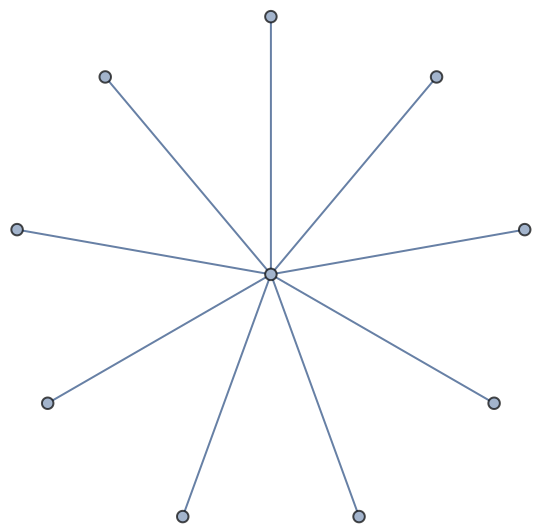In:=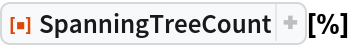Out=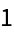A cycle on n vertices contains exactly n spanning trees, since deleting any edge creates a tree:

 In:=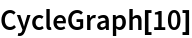Out=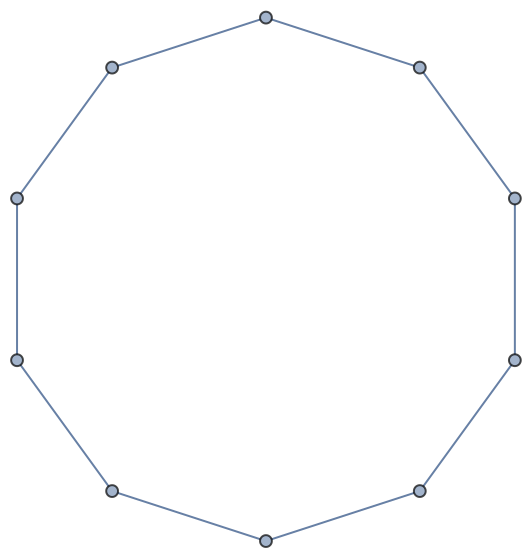In:=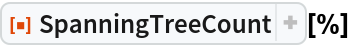Out=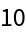The number of spanning trees of a complete graph is nn-2, as was proved by Cayley:

 In:=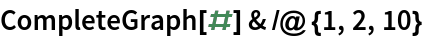Out=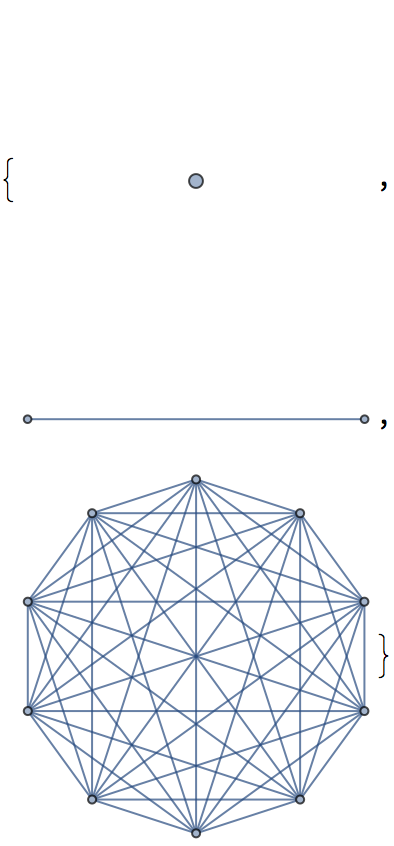In:=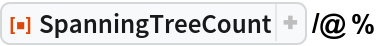Out=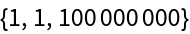In:=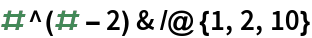Out=GraphData[name,"SpanningTreeCount"] gives the number of spanning trees for a named graph:

 In:=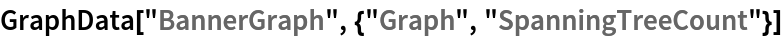Out=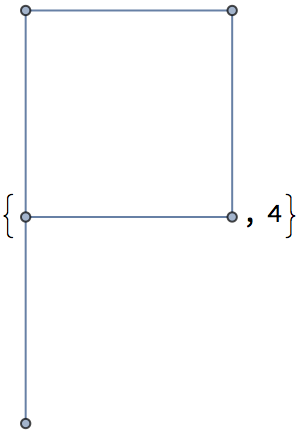In:=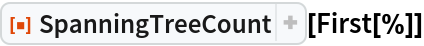Out=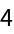In:=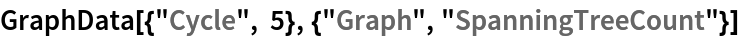Out=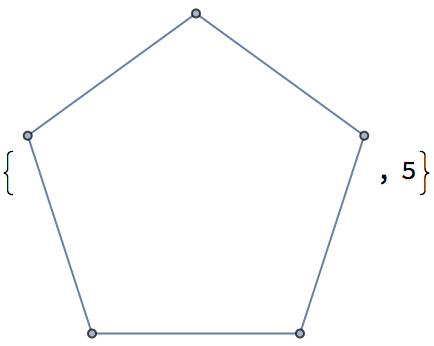In:=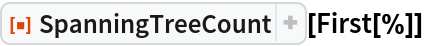Out=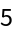## Version History

• 1.0.0 – 01 June 2020